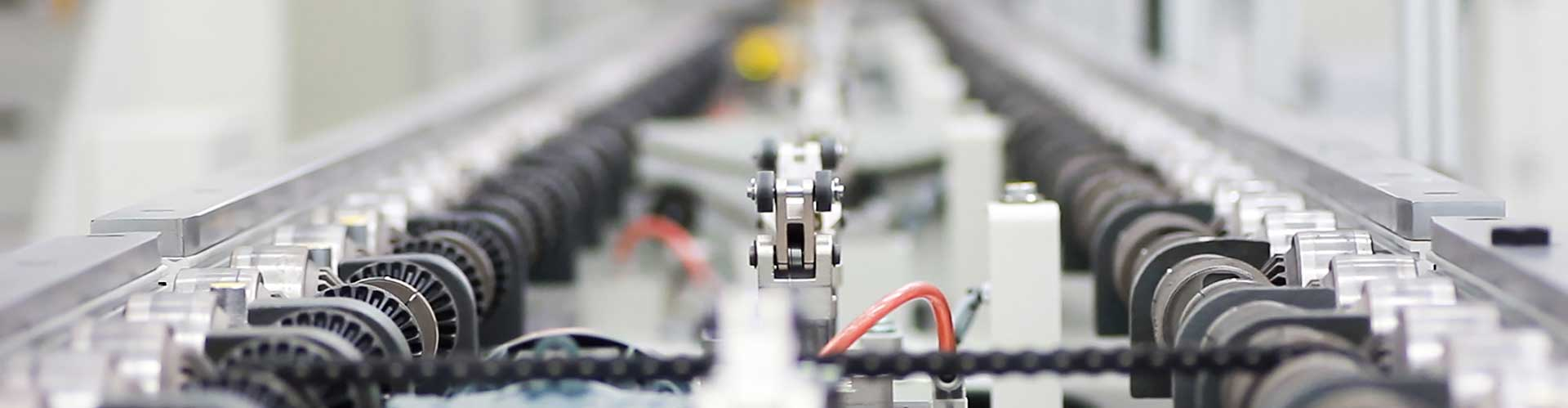﻿ FAQ - Shenzhen MICNO Electric Co., Ltd.# FAQ

All questions
• Q
1. What is a frequency inverter?
A

Answer: The inverter is a power control device that converts the power frequency to another frequency by using the on/off function of the power semiconductor device

• Q
2. How much energy does the inverter itself consume?
A

A: The power consumption of the inverter is 3-4%.

• Q
3. What are the control modes of the inverter?
A

Answer: V/F sinusoidal pulse width modulation mode, open loop vector control mode, closed loop vector control mode and direct torque control mode inverter.

• Q
4. Why does the voltage of the inverter change in proportion to the frequency?
A

Answer: In the case of variable frequency speed regulation, the magnetic circuit of the motor changes with a large range of operating frequency fX. It is very easy to seriously saturate the magnetic circuit of the motor, resulting in severe distortion of the excitation current waveform and high peak value. Peak current. Therefore, the frequency and voltage should be changed in proportion, that is, the frequency of the inverter is controlled while changing the frequency, so that the magnetic flux of the motor is kept constant to avoid the occurrence of weak magnetic and magnetic saturation.

• Q
5. What is the difference between frequency accuracy and resolution?
A

Answer: 1. Frequency accuracy refers to the accuracy of the inverter output frequency, that is, the error between the actual output frequency of the inverter and the given frequency. (The frequency accuracy is 0.01%)

The frequency resolution refers to the minimum adjustment amount of the output frequency, that is, the small difference between the output frequencies of each adjacent two gears (the frequency resolution is 0.01HZ)